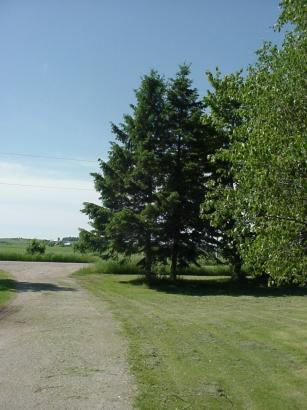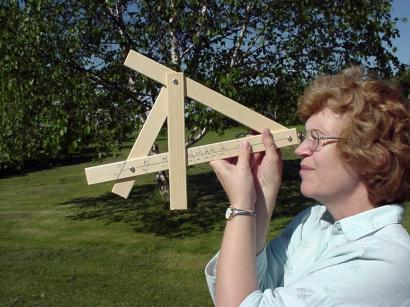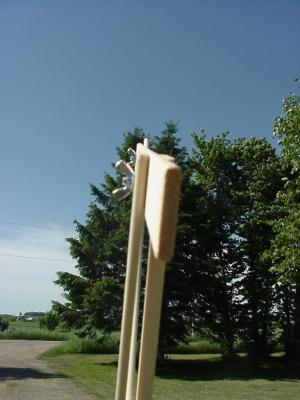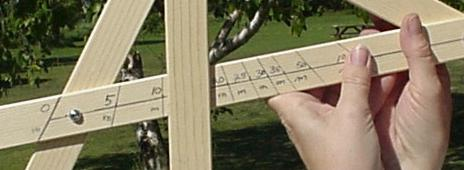Let's determine the height of this spruce tree.

Measure a distance of 20 meters back from the base of the tree.
If you do not have a tape measure, large paces are approximately 1 meter.Loosen the wing nut of the Pendulum Arm so it swings freely.
Look along the top of the Sighting Arm.
Align the Sighting Arm with the top of the tree.The height of the tree is indicated by the number along the edge of the Pendulum Arm.
The number indicated here is just over 10 meters - use 11 meters.
Assuming the ground is level, add your height to the number above to get a final number.
If your height is 1.5 m, the height of the tree would be 11m + 1.5m = 12.5 meters.

This device is calibrated to work at a distance of 20 meters from the object.
It does work at different distances but you must apply a correction factor.
ie. If you use it at a distance of 40 meters - 2 X 20
you would multiply the scale reading by 2.

For the engineers and mathemeticians reading this, the scale has been adjusted to
allow for the width of the pendulum arm.

Return